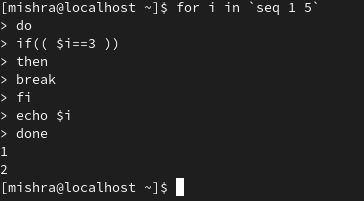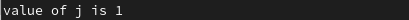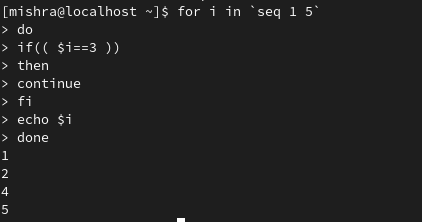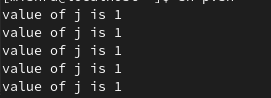Open in App
Not now

# Break and Continue Keywords in Linux with Examples

• Last Updated : 24 Dec, 2020

Both “break” and “continue” are used to transfer control of the program to another part of the program. It is used within loops to alter the flow of the loop and terminate the loop or skip the current iteration.

### break

The break statement is used to terminate the loop and can be used within a while, for, until, and select loops.

Syntax

```break [N]
// N is the number of nested loops.
// This parameter is optional.
// By default the value of N is 1.```

Using break command in a loop.It can be seen that when the value of i is 3, the loop execution is terminated and hence i is only printed upto 2.

Example: Using break with the value of N. Consider an example:

```for i in `seq 1 5`
do
for j in `seq 1 5`
do
if(( \$j== 2 ))
then
break 2
fi
echo "value of j is \$j"
done
echo "value of i is \$i"
done```

As the value of break is 2, when the value of j is 2, both the loops are exited in a single step. So, the only value of j is printed when it was 1.### continue

Continue is a command which is used to skip the remaining command inside the loop for the current iteration in for, while, and until loop.

Syntax:

```continue [N]
// the optional parameter N specifies the nth enclosing loop to continue from.
// This parameter is optional.
// By default the value of N is 1.```

Using break command in a loopExample: Using continue with the value of N. Consider an example:

```for i in `seq 1 5`
do
for j in `seq 1 5`
do
if(( \$j== 2 ))
then
continue 2
fi
echo "value of j is \$j"
done
echo "value of i is \$i"
done```

Continue skips both the loop when the value of j is 2 and hence, the code only executes when the value of j is 1.#### Difference between break and continue

My Personal Notes arrow_drop_up